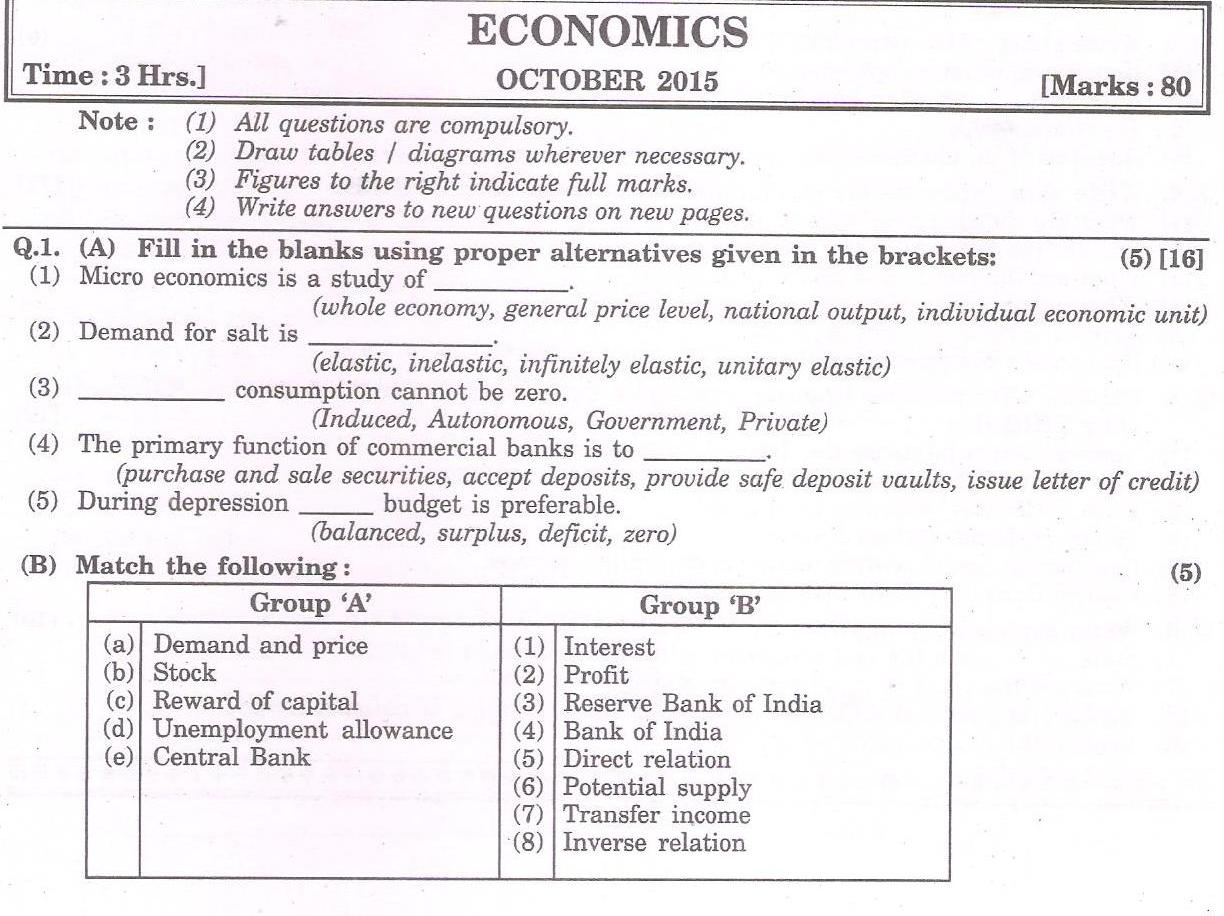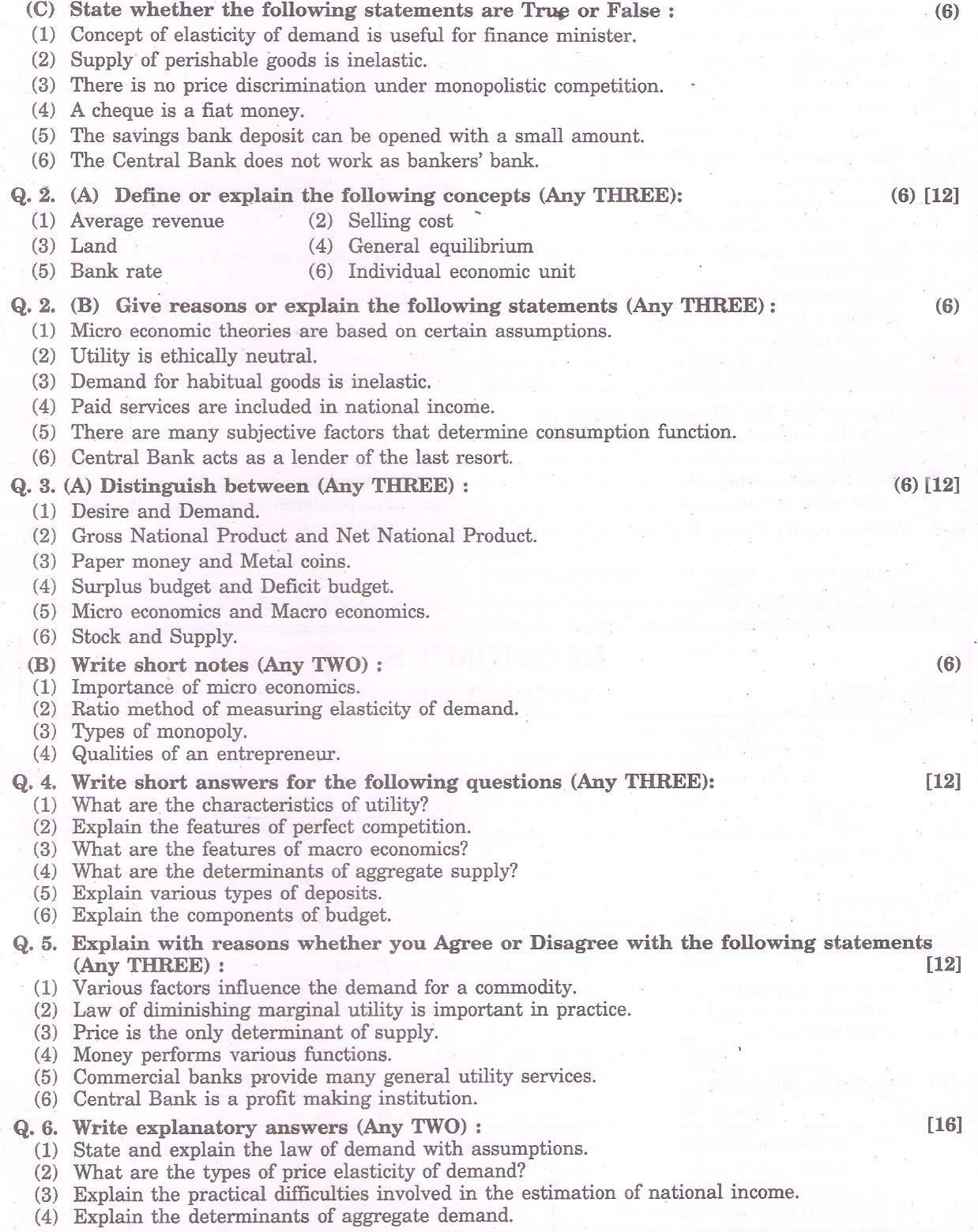### HSC Economics October 2015 Board PaperQ. 1. A. Fill in the blanks

1. Individual economic unit
2. Inelastic
3. Autonomous
4. Accept deposits
5. Deficit.

Q. 1. B. Match the following:

 Group A Group B a. Demand and Price (8) Inverse relation b. Stock (6) Potential Supply c. Reward of Capital (1) Interest d. Unemployment allowance (7) Transfer Income e. Central Bank (3) Reserve Bank of India

Q. 1. C. True or False

1. True
2. True
3. False
4. False
5. True
6. False

Q. 2. A. Define or Explain

(3) Land

(5) Bank Rate

Q. 2. B. Give reasons or explain the following statements. (Any Three) (6)

Q. 3. (A) Distinguish between (Any Three) (6)

Q. 3. (B) Write short notes. (Any Two) (6)

Q. 4. Write short Answer for the following questions. (Any Three) 

Q. 5. Explain with resons whether you ‘agree’ or ‘disagree’ with the following statements. (Any Three) 

Q. 6. Write explanatory answer: (Any Two) 

(1) State and explain the ‘Law of Demand’ with assumptions.